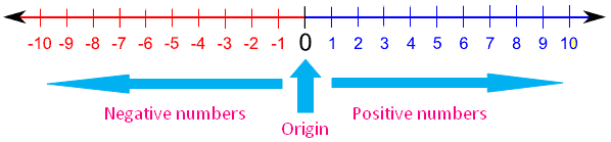# Addition of Integers Using the Number Line

## The Number LineA number line is a straight line with a "zero" point in the middle, with positive and negative numbers listed on either side of zero and going on indefinitely.

## Directions:

Move the sliders (red dot and green dot) to add two integers. Investigate what happens at the equation on the upper left. Observe the red and green arrows below.

How do we get the sum of two addends if both are positive?

What is the sign of the result if both addends are positive?

How do we get the sum of two addends if both are negative?

What is the sign of the result if both addends are negative?

How do we get the sum of two addends if one is positive and the other one is negative?

How do we determine the sign of two addends if one is positive and the other one is negative?

The number line just helped us to show what happens to the magnitude and to the sign of the result in adding integers. But what if we are going to add too large numbers is the number line still practical to use?Of course it is not. So, how will you add integers that are too large in magnitude?

Create a rule on adding integers without using the number line.